# Double-layer potential

(diff) ← Older revision | Latest revision (diff) | Newer revision → (diff)

An expression of the type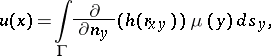(1)

whereis the boundary of an arbitrary bounded-dimensional domain,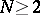, and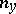is the exterior normal to the boundaryofat a point;is the potential density, which is a function defined on;is a fundamental solution of the Laplace equation: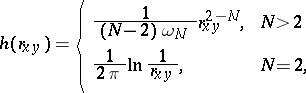(2)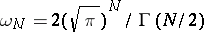is the area of the surface of the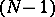-dimensional unit sphere, andis the distance between two pointsand. The boundaryis of class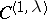; it is a Lyapunov surface or a Lyapunov arc (cf. Lyapunov surfaces and curves).

Expression (1) may be interpreted as the potential produced by dipoles located on, the direction of which at any pointcoincides with that of the exterior normal, while its intensity is equal to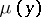.

If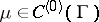, thenis defined on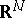(in particular, on) and displays the following properties.

1) The functionhas derivatives of all orders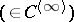everywhere in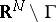and satisfies the Laplace equation, and the derivatives with respect to the coordinates of a point may be computed by differentiation of the integrand.

2) On passing through the boundarythe functionundergoes a break. Letbe an arbitrary point on; letandbe the interior and exterior boundary values; then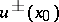exist and are equal to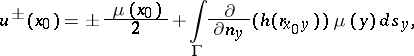(3)

and the integral in formula (3) as a function of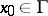belongs to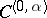for any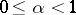; also, the function equal toinand toonis continuous on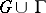, while the function equal toin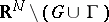and equal to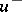onis continuous in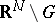.

3) If the density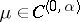and if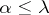, then, extended as in (2) onor, is of classinor in.

4) If, and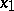and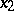are two points on the normal issuing from a pointand lying symmetric about, then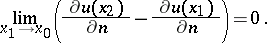(4)

In particular, if one of the derivatives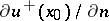,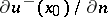exists, then the other derivative also exists and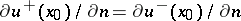. This is also true ifand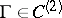.

The above properties can be generalized in various ways. The densitymay belong to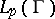,. Then,outsideand it satisfies the Laplace equation, formula (3) and (4) apply for almost-alland the integral in (3) belongs to.

The properties of double-layer potentials, regarded as integrals with respect to an arbitrary measuredefined on, have also been studied: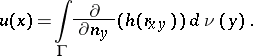Here, too,outsideand it satisfies the Laplace equation. Formulas (3) and (4) apply for almost-allwith respect to the Lebesgue measureafterhas been replaced by the density. In definition (1) the fundamental solution of the Laplace equation may be replaced by an arbitrary Lewy function for a general elliptic operator of the second order with variable coefficients, whileis replaced by the derivative with respect to the conormal. The properties listed above remain valid .

The double-layer potential plays an important role in solving boundary value problems of elliptic equations. The representation of the solution of the (first) boundary value problem is sought as a double-layer potential with unknown densityand an application of property (2) leads to a Fredholm equation of the second kind onin order to determine the function, . In solving boundary value problems for parabolic equations use is made of the concept of the thermal double layer potential, i.e. of an integral of the type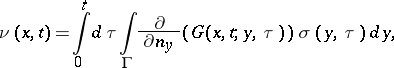where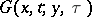is a fundamental solution of the thermal conductance (or heat) equation in an-dimensional space: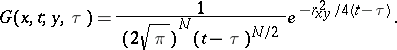Here,is the potential density. The functionand its generalization to the case of an arbitrary parabolic equation of the second order have properties which are similar to those described above for, , .

How to Cite This Entry:
Double-layer potential. Encyclopedia of Mathematics. URL: http://encyclopediaofmath.org/index.php?title=Double-layer_potential&oldid=16189
This article was adapted from an original article by I.A. Shishmarev (originator), which appeared in Encyclopedia of Mathematics - ISBN 1402006098. See original article